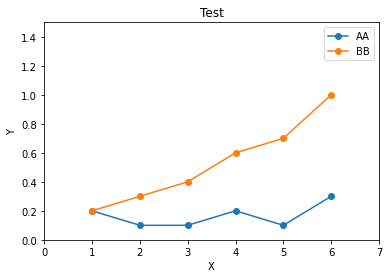► Python介紹

Python是一種易於學習、功能強大、直譯式並且物件導向的程式語言(由Guido van Rossum創造)，可執行於多平台，屬於通用型程式語言，Python 有幾項特點：

1. 語法簡潔：

Ｃ、Java及Android等語言，需要經過存檔、編譯及執行等三步驟，才能夠將你寫的程式碼執行運作，而Python不必存檔及編譯，寫完程式碼就可以直接執行，而且只需要輸入print(“Hello! World”)，就可以直接打印Hello! World，去除函示使用大括號（{}）的功能，以冒號（：）及換行table或四個空白替代大括號。

1. 功能強大：

Python可以用在深度學習、機器學習、網頁設計、手機 App 撰寫、遊戲程式設計、自動化控制、生物醫學、大數據…等領域，Python可以說是「萬用語言」。其他工程師撰寫好用 Python 程式打包成Library，允許你進行安裝使用﹐可以省下非常多的時間。

1. 跨平台：

Python支援各種主要的作業系統。

Python擁有完整的資料分析套件，常用的如下：

1.統計科學計算：Numpy、Scipy、Statsmodels。
2.結構化資料處理與分析：Pandas。
3.資料探索編輯器：Jupyter Notebook。
4.深度學習：TensorFlow、Pytorch。
5.大數據處理：PySpark。
6.機器學習：Scikit-Learn。

► Python基礎語法

Python的輸出指令就是print，只需要輸入print(‘Hello Word’)，直接執行就可以看到輸出結果Hello Word被打印出。

Python常見的基本變數有：int (整數)、float (浮點數)、str (字串)。不像其他語言需要先定義變數型態，在python的世界裡，會依照出史哲決定變數型態，常常忘記自己設定的變數型態可以使用type(變數) 這個指令做查看。

 運算子 功能 x + y X加Y x - y X減Y x * y X乘Y x / y X除以Y x // y X除以Y，只取整數解 x % y 求X除以Y的餘數 x ** y X的Y次方

``a = ‘1234567\89012345’print(a)​``

s = ‘Hello’
s[1:] 取得從第一個索引之後的全部元素
s[:3] 取得從0到索引為第3個元素
s[:] 取得所有元素
s[::1] 類似For迴圈，從0開始，每次遞增1
s[::2] 類似For迴圈，從0開始，每次遞增2
s[::-1] 類似For迴圈，從0開始，每次遞減1

Python 的 List 比較接近於 Java 的 ArrayList的使用，但是Python的List內可以放不同類型的物件，以中括號表示：
``my_list=[1,2,3]my_list=['A string',23,100.232,'o']​``

`` hello=list('Hello world')``

print(hello)
['H','e','l','l','o',' ','w','o','r','l','d’]

``dic={'a':100, 'b':"yes", 'c':0.98}``

Tuple跟List很像，但不一樣的地方在於Tuple內的值無法被修改，建立完成後就不可被更動，Tuple有幾個優點，第一個為佔用空間比List少，第二個為可當作Dictionary的key，第三個為當做函式引數，Tuple以小括號表示：
``tuple = (1,2,3)​``

``if True:    print('It was true!')​``

For迴圈

``a = [1,2,3,4,5,6,7,8,9,10]b = ""for num in a:  b = b + str(num)print(b)​``

``def addNum(a, b):        return a+bprint(addNum(5, 6))​``

► Numpy套件基本介紹

NumPy(Numerical Python) 是Python 語言的一個擴展函式庫，支持大量的維度數組與矩陣運算，此外也針對數組運算提供大量的數學函數庫。

NumPy 通常與SciPy（Scientific Python）和Matplotlib（繪圖庫）一起使用，有助於通過Python 學習數據科學或者機器學習。

``na=np.array([1,2,3,4,5]) #一維陣列，跟一般List操作類似na=np.array([[1,2,3,4,5],[6,7,8,9,10]]) #二維陣列``

SciPy 包含的模塊有最優化、線性代數、積分、插值、特殊函數、快速傅里葉變換、信號處理和圖像處理、常微分方程求解和其他科學與工程中常用的計算，只需要做安裝引用就可以實現這些數學運算。

Matplotlib 是Python 編程語言及其數值數學擴展，可視化操作界面，操作如下：

``import numpy as npimport matplotlib.pyplot as pltx=np.arange(1,7)y=[0.2,0.1,0.1,0.2,0.1,0.3]cdf=np.cumsum(y)plt.plot(x,y, marker="o",label="AA")plt.plot(x,cdf,marker="o",label="BB")plt.xlim(0,7)plt.ylim(0,1.5)plt.xlabel("X")plt.ylabel("Y")plt.title("Test")plt.legend()plt.show()``★博文内容均由个人提供，与平台无关，如有违法或侵权，请与网站管理员联系。

★文明上网，请理性发言。内容一周内被举报5次，发文人进小黑屋喔~